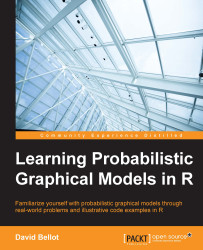•#### Learning Probabilistic Graphical Models in R#### Overview of this book

Probabilistic graphical models (PGM, also known as graphical models) are a marriage between probability theory and graph theory. Generally, PGMs use a graph-based representation. Two branches of graphical representations of distributions are commonly used, namely Bayesian networks and Markov networks. R has many packages to implement graphical models. We’ll start by showing you how to transform a classical statistical model into a modern PGM and then look at how to do exact inference in graphical models. Proceeding, we’ll introduce you to many modern R packages that will help you to perform inference on the models. We will then run a Bayesian linear regression and you’ll see the advantage of going probabilistic when you want to do prediction. Next, you’ll master using R packages and implementing its techniques. Finally, you’ll be presented with machine learning applications that have a direct impact in many fields. Here, we’ll cover clustering and the discovery of hidden information in big data, as well as two important methods, PCA and ICA, to reduce the size of big problems.
Learning Probabilistic Graphical Models in RCreditswww.PacktPub.comPrefaceFree Chapter
Probabilistic ReasoningExact InferenceLearning ParametersBayesian Modeling – Basic ModelsApproximate InferenceBayesian Modeling – Linear ModelsProbabilistic Mixture ModelsAppendixIndex## Chapter 2. Exact Inference

After building a graphical model, one of the main tasks one wants to perform is putting questions and queries to the model. There are many ways to use graphical models and the representation they give from a joint probability distribution. For example, we can study interactions between random variables. We can also see if any correlation or causality is captured by the model. Moreover, as probability models governing the random variables are parameterized, it means that their probability distribution is fully known through being familiar with some numerical parameters. We might be interested in knowing the values of those parameters when other variables are observed.

The main focus of this chapter is on introducing algorithms to query a distribution that uses the model and observations on a subset of variables in order to discover the posterior probability distribution on another subset. It is not necessary to observe and query all the variables. In fact, all the...